# Supertiles.

## A. 2×2 integer edge sequence solution.

The smallest first generation supertile is one based on either the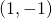or the (,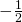) edge sequence.  In fact, the latter may be considered to be a special case of the first and will be discussed later. The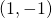edge sequence means that there is only one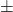pair of edge angles, or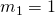. It follows that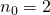and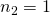, i.e. the supertile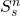has to be constructed from the set of prototiles {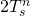,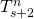,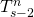}. In our general model the arrangement of the tiles is given by the supertile matrix

(1)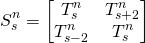In order to tile the plane, the inflation factor should be larger than one. Because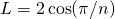,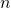should be larger than three. Below, supertiles are shown for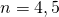and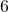. Rhomb prototiles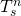and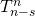are identical objects, but supertilesand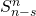are not. The supertiles also loose the twofold rotation axis perpendicular to the plane of the prototiles. Consequently, after the first generation supertiles there are four different substitution rules for the next supertile generation, which we have denoted by a), b), c) and d). a) is the basic supertile, in b) the tiles of a) have been rotated over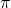, in c) the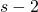and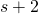tiles of case a) have been replaced by the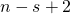and the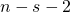tiles respectively, and in d) the tiles of case c) have been rotated over. In matrix notation:

(2)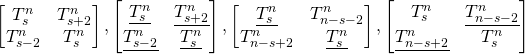Underlined entries denote prototiles rotated over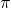.

Fig. S1-S3 Substitution tiles for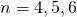.

The supertiles consist only of single positive and negative parts, i.e. the tile overlaps which arise during the tiling proces are exactly compensated by negative tiles or tile parts. The first generation supertiles consist of two prototiles, one rotated over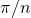and the other over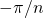and aandprototiles in the original position as drawn in the pictures. Negative tiles play a part for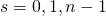and. For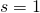the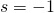negative tile cuts of pieces of the other three tiles, as is illustrated in the picture for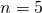below. For the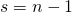supertile, on the other hand, the two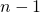prototiles partly overlap. This overlap is removed by the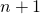prototile.  The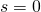supertile is just a zigzag line because the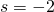annihilates the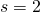tile. This is best seen by starting with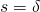,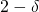and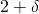prototiles,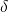being a small value  approaching zero. The negativeand the positiveprototile do not overlap in the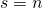supertile, although the total area is zero as it should.

## B. 2×2 half-integer edge sequence solution.

Looking at the first three figures it becomes  clear that for substitution rules a) and b) there are two independent prototile sets for even and odd s. This is also true for substitution rules c) and d) ifis even. In fact, the half-integer edge sequence solution is identical to the integer edge sequence solution for a doubled-value and even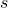. We will discuss these solutions in more detail below.

The (,) edge sequence means that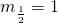. It follows thatand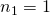, i.e. the supertilehas to be constructed from the set of prototiles {,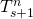,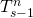}. In our general model the arrangement of the tiles is given by the supertile matrix

(3)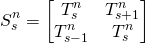Below the first two generations of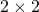supertiles forwith a halfinteger edge sequence are shown. If one compares these tiles with the integer case it is clear that negative tiles play a smaller role. Only for substitution rule a) a negative part appears in thetile. For substitution rule c) and d) the tiles are not even cut up. The only tile having a negative part is thetile. This tile is only involved in the construction of next generationandtiles. In the latter case, its role is to move a patch of complete tiles from one end of the supertile to another, also for higher generations.

a) Basic (1, -1) rhomb tiling,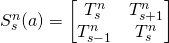b) Koch (1, -1) rhomb tiling,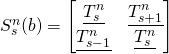. The supertile edges are related to the so called Koch curves.
c) Lancon Billard (1,-1) rhomb tiling,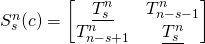and its mirror image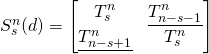.  Forthe even s first generation substitution tiles are the Lancon Billard substitution tiles.